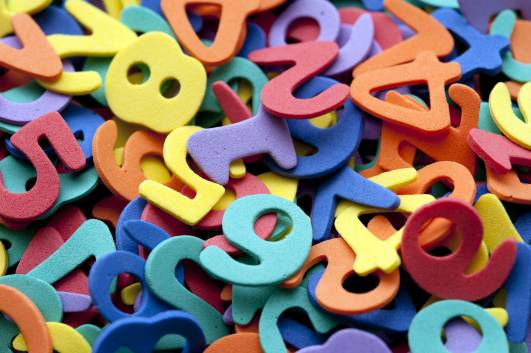# How To Convert 5.6 Into A Fraction?

5.6 converted into a fraction is 5 and 3/5. It's pretty simple to work out once you know how!As far as 5.6 is concerned, forget about the 5 for the moment and concentrate on the 0.6. The 6 is in the first decimal place, which is for tenths of a whole number. 0.6 is therefore the same as six-tenths or 6/10. We could write 5 and 6/10, but as 6 and 10 are both divisible by 2, we can simply 6/10 to 3/5, or three-fifths. This leaves us with 5 and 3/5.

Converting a Mixed Number to an Improper Fraction

If you meant changing 5.6 to an improper fraction rather than a mixed number (5 and 3/5 is a mixed number), we can do that as well.

To do this, one must multiple the denominator (the bottom number of a fraction) by the whole number. In this instance, we are times 5 (denominator) by 5 (the whole number). We then add the total to the numerator (the top number of a fraction). This gives us (5 x 5) + 3 = 28.

We retain the denominator for improper fractions. This gives us twenty-eight fifths or 28/5.

Here's more on converting numbers into fractions:

thanked the writer.